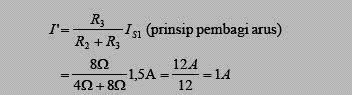## Tuesday, July 21, 2009

### Teorema Superposisi untuk Analisi Rangkaian DC

Teoerma This states that the size of the current or voltage in an electrical element in the series can be determined by considering the contribution from each source independently. At the time we consider the contribution from a source other then the source eliminated. Thus eliminated voltage source (V = 0) into a series of short relationships. While to eliminated current source (I = 0) into a series of open relationship. Large currents or voltages in the series who want to search the Answer algebraically from current or voltage due to contributions from each source. With these theorems we shall consider the flow direction or polarity of the voltage at the figure donations from each source.

• Example
By using the theorems superposisi, specify the size of the flow (I) through the R2 in the following series!Taking account of Cash from the voltage source E1, the source voltage E2 to be eliminated so that a series of short relationships, such as the image below:By using the principle Flow distributor, illicit flow of E1 can search on:Taking account of Flow E2 from the voltage source, voltage source E1 to be eliminated so that a series of short relationships, such as the image below:By using the principle Flow distributor, illicit flow of E2 can search on:So: Flow through a total reaktansi Answer R2 is a contribution of each source (cash donation dri E1 and E2) that is equal to:
I = I '+ I "= 1A = + 0.25 A 1.25 A

Skema Rangkaian Elektronika